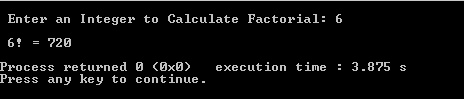### C Code Snippets 3 - Factorial Calculation (Iterative & Recursive)

Hello everyone, in this post I am going to share flow charts and source codes on how to calculate a factorial by using iterative and recursive functions. I think the flow charts and the codes are self-explanatory. If you have any questions do not hesitate to write a comment below.

``````
/**
* Author : Berk Soysal
*/

#include <stdio.h>

int main()
{
int i,factorial=1,number;

// User Input
printf("Enter a number:");
scanf("%d",&number);

if(number<0)
{
printf("\nPlease Enter Zero or a Positive Integer !\n");
}
else
{
// Calculate the Factorial
for(i=1; i<=number; i++)
factorial=factorial*i;

// Print the Result
printf("\n%d! = %d\n",number,factorial);
}
return 0;
}

``````

ResultRecursive Factorial Calculation

Recursion is a technique in which a function calls itself, for example our code below in the factorial function is calling itself until num reaches 0. To solve a problem using recursion you must first express its solution in recursive form.
` `

``````
/**
* Author : Berk Soysal
*/

#include<stdio.h>

//Function Prototype
long factorial(int);

//Main function
int main()
{
int num;

// User Input
printf("\n Enter an Integer to Calculate Factorial: ");
scanf("%d", &num);

if (num < 0)
printf("\n Please Enter Zero or a Positive Integer !\n");
else
{
printf("\n %d! = %ld\n", num, factorial(num));
}

return 0;
}

//Recursive Factorial function
long factorial(int num)
{
if (num == 0)
return 1;
else
return(num * factorial(num-1));
}

``````
```
```
```
Result```
```
```
```
```
```Keywords: CCode Snippets
```